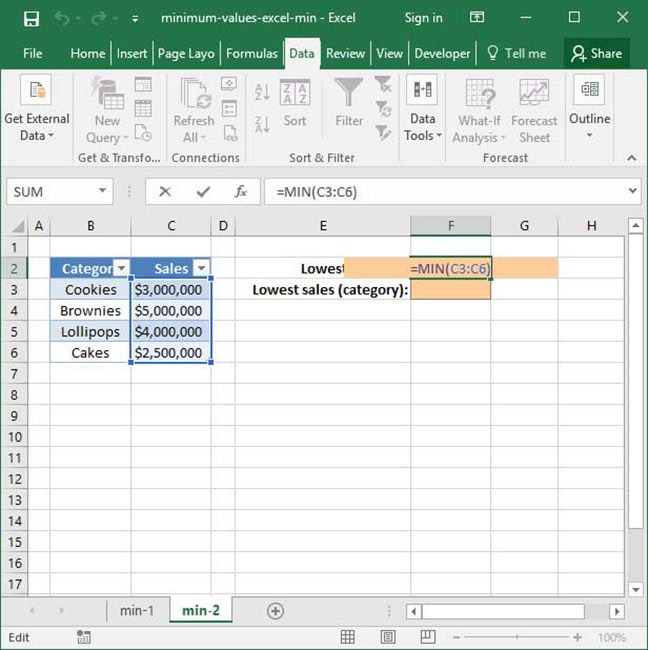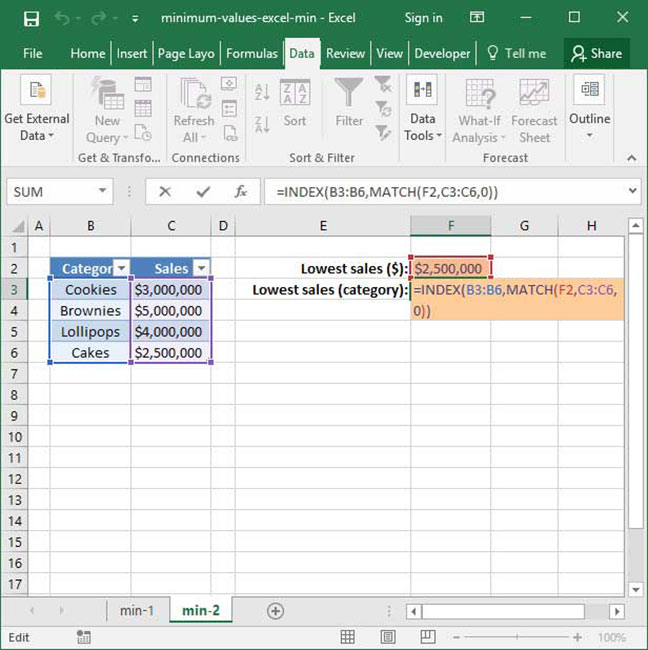# Minimum Values in Excel Using MIN

If you've studied the MAX function, you know that there's an easy way to find the maximum value in a range or series of numbers. But what if you want to find the minimum value?

It turns out that finding minimum values is easy with Excel's MIN function. MIN works just like MAX — it takes a series of numbers or a cell range as an input, then evaluates everything inside and spits out the minimum value it sees.

Keep reading to find out more about how to use the MIN function.

## A simple use of the MIN function

Like MAX, MIN is a very simple function. Its syntax is as follows:

`=MIN(number_or_range_1, number_or_range_2 (optional)...)`

There are a couple of things here to be aware of: first, MIN accepts either a series of numbers, or a range of cells as its arguments. Second of all, it can take an unlimited number of arguments. Like MAX, it will evaluate all of its arguments and spit out only the number that is smallest amongst them.

Here's MIN in action:

`=MIN(6, 7, 8)Output: 6`

The value output here is 6, because 6 is the smallest argument provided to the function.

Here's another example with a more extensive numerical range:

`=MIN(-45, 0, -10, 109, 76)Output: -45`

The output here is -45, because -45 is the minimum value provided in the formula.

## MIN as part of a cell range

Like MAX, MIN can be used as part of a large range of sells rather than a series of manual inputs. Consider the following example:`=MIN(C3:C6)Output: \$2,500,000`

Above, we test the MIN function on the range C3:C6 to find the lowest value contained within its cells. The output of the formula is \$2,500,000, because \$2,500,000 is the minimum value found in the range provided.

As with the MAX formula, we can also combine MIN with INDEX MATCH to find the name of the category with the lowest sales. Take, for example, the following formula:`=INDEX(B3:B6, MATCH(F2, C3:C6, 0))Output: "Cakes"`

If you want to learn more about how INDEX MATCH works, check out our INDEX MATCH primer.

## When else can we use MIN?

The MIN function is incredibly versatile; it can be used to find minimum values in a wide range of scenarios. The following are just a few examples of why you might want to incorporate MIN into your spreadsheets:

• Find the first date in a given range;
• Identify lowest-performing students by assignment to see who needs additional support;
• Identify salespeople who have underperformed the average in a given period of time;
• Search for temperature minimums over time; or
• Find stock price lows.